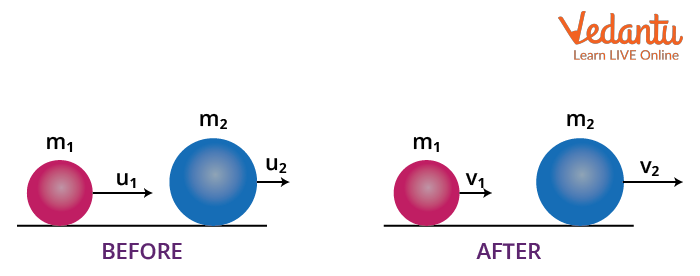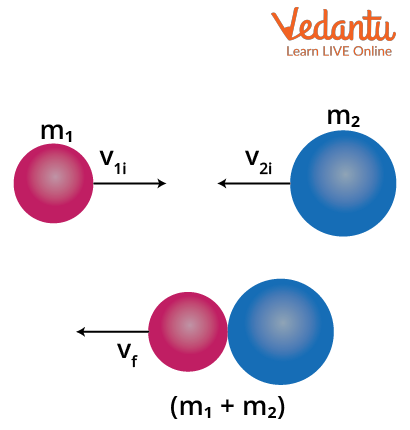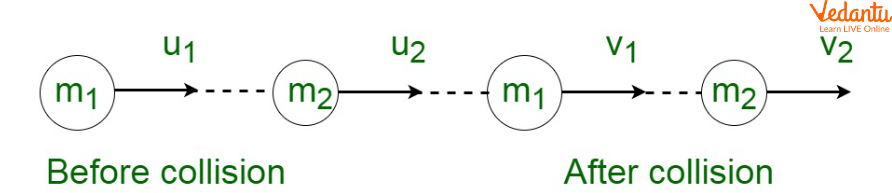Courses
Courses for Kids
Free study material
Free LIVE classes
More

# Collision - Important Concepts and Tips for JEELIVE
Join Vedantu’s FREE Mastercalss

## What is Collision?

When two moving bodies interact and experience a sudden impact force during their motion, then this event is known as a collision. During the impact, both bodies exert a certain amount of force on each other. This force is known as collision force.  Due to the collision, both the bodies change their momentum and energy. In the collision, the determination of velocities can be done with the help of the law of conservation of momentum and the law of conservation of energy.

A collision between two bodies can occur by making actual physical contact and sometimes they don’t make any physical contact with each other but a collision occurs. A collision can be perfectly elastic, inelastic, and perfectly inelastic. In this article, an attempt has been made for giving brief information (like defining collision, defining elastic and inelastic collision, what causes a collision, collision force, types of collisions, line of impact in a collision, etc.).

## What Causes Collisions?

When the collision happens between the two bodies, they interact with each other with a sudden impact force for a shorter duration of time. The major causes due to which the collision occurred between two bodies are given below:

• For the collision to happen, the line of the impact of these colliding bodies must be collinear or intersect with each other. The line of impact is the line that passes through the centre of the colliding bodies. If two moving bodies have a common line of impact or intersecting line of impact, then the collision will occur.

• When the line of impact is common between the moving bodies and if the velocity of the first body is greater than the second body, then there will be chances of collision and it might be another cause of the collision.

## Types of Collisions

The types of collision are differentiated on the basis of the conservation of energy principle and the direction of colliding bodies before the collision.  The types of collisions are categorised under two category which is shown below:

### 1. Based on The Conservation of Energy Principle

In this section, the types of collisions are differentiated based on the law of conservation of energy and conservation of momentum during the collision. There are three types of collisions that are shown below:

• Perfectly elastic collision

• Inelastic collision

• Perfectly inelastic collision

### 2. Based on The Direction of Colliding Bodies

In this section, the types of collision depend upon the direction of the colliding bodies before the collision takes place. Based upon that, the collision is of two types:

• One-dimensional collision (or Head-on collision)

• Oblique collision

## Elastic Collision

In an elastic collision, when a collision takes place between two bodies, then the kinetic energy of two bodies will remain the same. A perfectly elastic collision is an ideal case of elastic collision. For perfectly elastic collision, the value of the coefficient of restitution is one. An example of perfectly elastic collisions is the bounce-back tendency of the ball when it hits the floor.Elastic collision

In a perfectly elastic collision, when two bodies collide with each other, then there is no change occurring in the total kinetic energy of the system. It means that there is not any transformation of energy occurring in the form of heat, noise, etc. It is a special case of elastic collision.

The collision formula of perfectly elastic collision is shown below:

In the given figure (image 1), the first body of mass m1 with velocity u1 strikes the second body of mass m2 moving with velocity u2. After the collision, the first body moves with v1 and the second body moves with v2. As the total momentum is conserved, then the initial momentum of both the bodies P1+P2 will be equal to the final momentum of both bodies P1’+P2.

$p_{1}+p_{2}=p_{1}^{\prime}+p_{2}^{\prime}$

The momentums will be,

\begin{align} &p_{1}=m_{1} u_{1} \\ &p_{2}=m_{2} u_{2} \\ &p_{1}^{\prime}=m_{1} v_{1} \\ &p_{2}^{\prime}=m_{2} v_{2} \end{align}

$m_{1} u_{1}+m_{2} u_{2}=m_{1} v_{1}+m_{2} v_{2}$ …..(1)

Also for an elastic collision the ratio of the relative velocity before and after collision is equal to 1. This means that,

\begin{align} &\dfrac{v_{2}-v_{1}}{u_{2}-u_{1}}=1 \\ &v_{2}-v_{1}=u_{2}-u_{1} \\ &v_{2}+u_{2}=v_{1}+u_{1} \end{align}

We can then write,

\begin{align} &v_{2}=v_{1}+u_{1}-u_{2} \\ &v_{1}=v_{2}+u_{2}-u_{1} \end{align}

Substituting the expression for v2 in equation (1) gives,

$m_{1} v_{1}+m_{2}\left(v_{1}+u_{1}-u_{2}\right)=m_{1} u_{1}+m_{2} u_{2}$

$v_{1}\left(m_{1}+m_{2}\right)=m_{1} u_{1}+m_{2} u_{2}+m_{2} u_{2}-m_{2} u_{1}$

$v_{1}\left(m_{1}+m_{2}\right)=u_{1}\left(m_{1}-m_{2}\right)+2 m_{2} u_{2}$

$v_{1}=u_{1} \dfrac{\left(m_{1}-m_{2}\right)}{\left(m_{1}+m_{2}\right)}+2 u_{2} \dfrac{m_{2}}{\left(m_{1}+m_{2}\right)}$

Similarly the expression for v2 will be,

$v_{2}=2 u_{1} \dfrac{m_{1}}{\left(m_{1}+m_{2}\right)}+u_{2} \dfrac{\left(m_{2}-m_{1}\right)}{\left(m_{1}+m_{2}\right)}$

These are the expressions for final velocities.

And there is no loss in total kinetic energy of the bodies since it is an elastic collision, therefore initial kinetic energy of both bodies (K.E) will be equal to the final kinetic energy of both the bodies (K.E).

\begin{align} &(K . E)=(K . E) \\ \\ &\dfrac{1}{2} m_{1} u_{1}^{2}+\dfrac{1}{2} m_{2} u_{2}^{2}=\dfrac{1}{2} m_{1} v_{1}^{2}+\dfrac{1}{2} m_{2} v_{2}^{2} \end{align}

## Inelastic Collision

In an inelastic collision, when two bodies collide with each other, then there will be some loss in the kinetic energy of the body. In this case, the body gets stuck to each other which indicates that one of the bodies has lost its kinetic energy.An Inelastic collision

An inelastic collision is a type of collision in which the kinetic energy is not conserved but the momentum remains conserved. In the given case, the value of the coefficient of restitution lies between 0 to 1. An example of inelastic collisions is dropping a ball of clay on the floor.

The collision formula for the inelastic collision is shown below:

In the given figure (image 2), the first body of mass m1 with velocity v1 strikes the second body of mass m2 moving with velocity v2. After the collision, the first body and second body move with velocity v. As the total momentum is conserved, then the initial momentum of both the bodies P1+P2 will be equal to the final momentum of both bodies P’1+P’2

\begin{align} &p_{1}+p_{2}=p_{1}^{\prime}+p_{2}^{\prime} \\ \\ &m_{1} v_{1}+m_{2} v_{2}=m_{1} v_{1}^{\prime}+m_{2} v_{2}^{\prime} \end{align}

Since the final velocity of both the bodies are same, then the formulation will be done as,

\begin{align} &v_{1}^{\prime}=v_{2}^{\prime}=v \\ &m_{1} v_{1}+m_{2} v_{2}=m_{1} v+m_{2} v \\ &\frac{m_{1} v_{1}+m_{2} v_{2}}{m_{1}+m_{2}}=v \end{align}

A perfectly inelastic collision is a special case of inelastic collision. In this type of collision, when two bodies collide with each other, then both of the bodies get to stick together and move further as a single body. In this case, a large change in kinetic energy is recorded. The value of the coefficient of restitution is 0. The collision formula will be the same as inelastic collision. An example of perfectly inelastic collisions is dropping a ball of clay on the floor.

## One Dimensional (or Head-on) Collision

One-dimensional collision or head-on collision is a case of elastic collision when two bodies move along the same path and the velocity of the first body is greater than the second body collides with each other and after the collision, they move with different velocities. The path of both moving bodies is the same as they move along the straight line joining their centre bodies.In this collision, the first body strikes the second one and has a head-on collision with the second body. In this encounter, the total kinetic energy of both bodies after the collision will be equal to the kinetic energy of the bodies before the collision which confirmed that there is no conversion of kinetic energy into other forms. An example of a head-on collision is when a car strikes any stationary objects like a tree.

## Oblique Collision

Oblique collision is a type of collision where the first body strikes the other one at an angle before the collision and after the collision, both of the bodies move with different velocities.Oblique collision

In the collision, the line joining the centre of both moving bodies is not the same and their respective line along the centre of the body intersect with each other at an angle. It also belongs to an elastic collision. If both bodies remain in the same plane before and after the collision, then it will be a two or three dimension collision.

The bodies are moving in the x direction initially so the initial momentum of the bodies in y direction will be zero. The momenta will be conserved in both the x and y directions. The conservation of momentum in the x direction will give,

\begin{align} &m_{1}{u_{1 x}}+m_{2} u_{2 x}=m_{1} v_{1 x}+m_{2} v_{2 x} \\ \\ &m_{1} u_{1}+m_{2} u_{2}=m_{1} v_{1} \cos \theta+m_{2} v_{2} \cos \phi \end{align}

Similarly for the y direction the law of conservation of momentum will give,

\begin{align} &m_{1} u_{1 y}+m_{2} u_{2 y}=m_{1} v_{1 y}+m_{2} v_{2 y} \\ \\ &m_{1} \cdot 0+m_{2} \cdot 0=m_{1} v_{1 y} \sin \theta+m_{2} v_{2 y} \sin \phi \\ \\ &m_{1} v_{1} \sin \theta=-m_{2} v_{2} \sin \phi \end{align}

The coefficient of restitution is the ratio of the final and initial relative velocities. For the x direction this coefficient of restitution can be written as,

\begin{align} &e=\dfrac{v_{2 x}-v_{1 x}}{u_{2 x}-u_{1 x}} \\ \\ &e=\dfrac{v_{2} \sin \phi-v_{1} \sin \theta}{u_{2}-u_{1}} \end{align}

These expressions can be used to find the initial as well as final velocities if the parameters are given in the question.

## Conclusion

This article concludes with brief information regarding the concept of collision. The major focus of this article is on the causes of collision, types of collision and defining the concept of line of impact. It also discussed the topics of the elastic and inelastic collision along with the collision formula. For validating and deriving the collision formula, it shares glimpses of the law of conservation of energy and momentum. It also classified the types of collision based on the direction of colliding bodies.

It gives brief information about topics (like defining collision, defining elastic and inelastic collision, what causes a collision, collision force, types of collisions, line of impact in a collision, etc.). This article also defines the concept with diagrams and equations of the different types of collision for a better understanding.

Last updated date: 26th Sep 2023
Total views: 2.4k
Views today: 0.02k

## FAQs on Collision - Important Concepts and Tips for JEE

1. Explain the concept of collision.

In a collision, when two objects come in contact with each other physically or non-physically, then the motion of the objects gets affected due to the forces exerted by them on each other. Then this event is known as a collision. Both objects experience a change in their momentum and energy resulting in a change of their respective velocities after the collision. The magnitude of the object’s velocities will be determined by the help of the law of conservation of momentum and the law of conservation of energy.

2. Why is it necessary to study the concept of collision in physics?

The concept of collision is used in physics for the understanding of the properties of atomic and subatomic particles of the material.  In physics, a device named the particle accelerator which is used for determining the atomic properties of the material by processing a controlled collision among the particles. The concept of collision is also used in the automobile industry for determining the safety aspects of the automobile in case of any fault or tragedy. Hence, it is necessary to study and analyse the concept of collision.

3. Does collision hold weightage in the JEE exam?

The conceptual knowledge about the collision is very important for the point of view. It is found to be one of the most vital topics in the preparation for the jee exam. Important topics such as centre of mass, collision, oblique collision, coefficient of restitution found many numerical in the previous year of JEE exam. This section has a weightage of nearly 2-5 percent and the number of questions can be expected to be 4-5 as per previous year JEE exam.